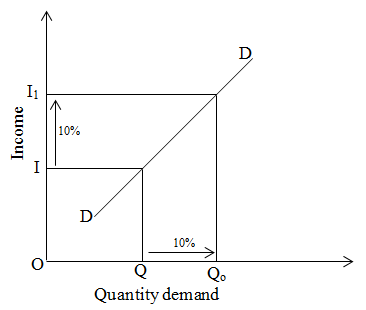# Income elasticity of demand equal to one

If the percentage change in quantity demand is equal to percentage change in income is known as income elasticity of demand equal to one. We can explain on the basis of the given figure:On the above figure, initial income is OI and quantity demand is OQ. When income is increased from I to I1 by 10% then the quantity demand is also increased from Q to Q1 by 10%.